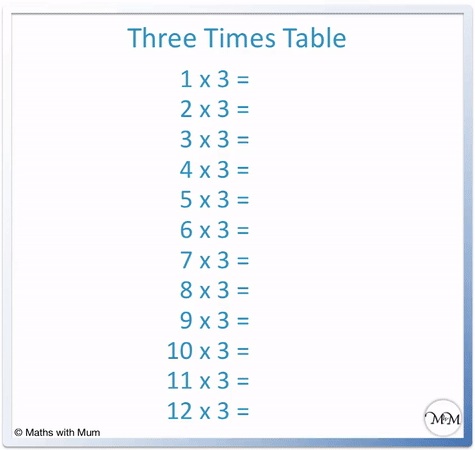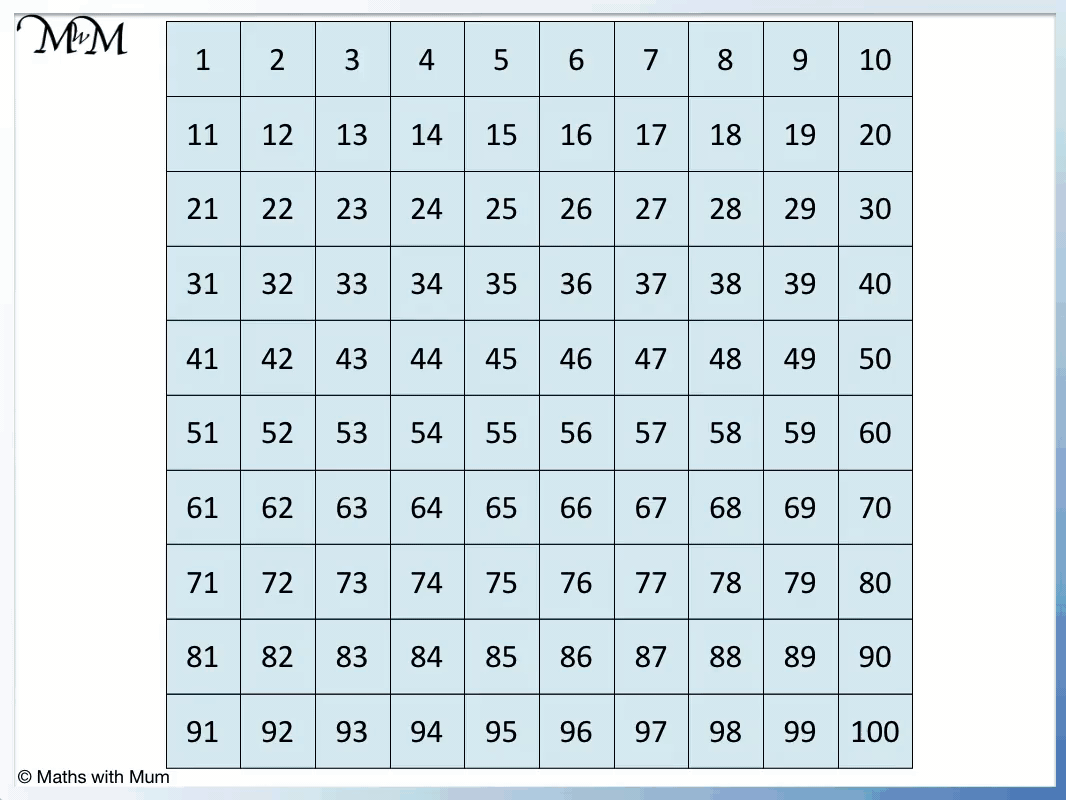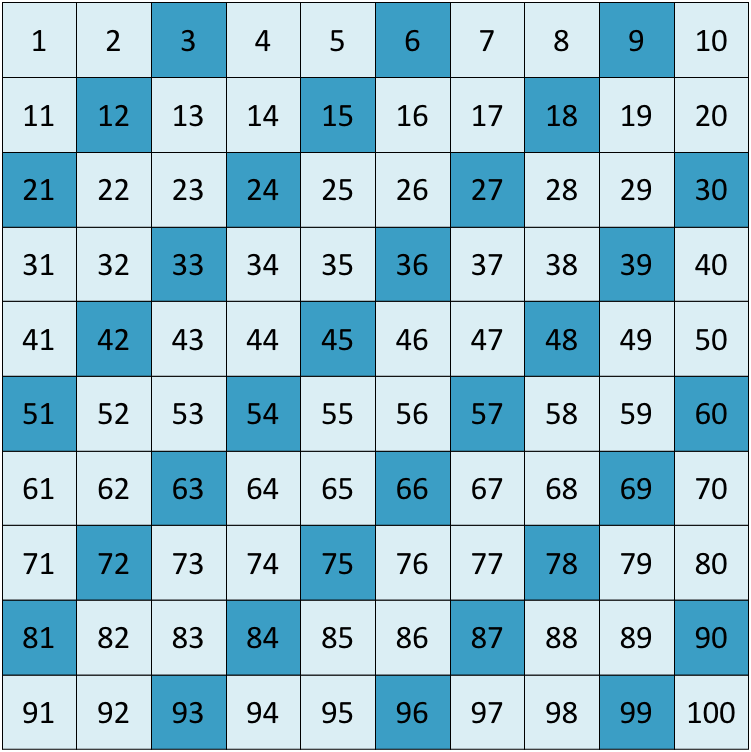# Multiples of 3

Multiples of 3• The multiples of 3 are numbers that can be divided exactly by 3, leaving no remainder.
• The answers to the three times table are the first few multiples of 3.
• For example 5 × 3 = 15 and so, the fifth multiple of three is 15.
• For example 100 × 3 = 300 and so, the hundredth multiple of 3 is 300.
• To test if a number is a multiple of 3, add its digits together. If the result is a multiple of 3, then the original number is also a multiple of 3.
• For example, 54 is a multiple of 3 because 5 + 4 = 9 which is also a multiple of 3.

A multiple of 3 is any number that can be divided by 3 exactly, leaving no remainder.

The first few multiples of 3 are seen in the 3 times table.#### Multiples of 3 Flashcards

Click on the multiples of 3 flashcards below to memorise the 3 times table:

×
×

=#### Multiples of 3: Interactive Questions

Multiples of 3: Interactive Questions

# Multiples of 3

## What are Multiples of 3?

Multiples of 3 are numbers that can be divided exactly by 3 with no remainder. Any whole number multiplied by 3 makes a multiple of 3. For example, 100 × 3 = 300 and so, 300 is the hundredth multiple of 3.

Here is a poster showing the 3 times table and the first twelve multiples of 3:• 1 × 3 = 3.
• 2 × 3 = 6.
• 3 × 3 = 9.
• 4 × 3 = 12.
• 5 × 3 = 15.
• 6 × 3 = 18.
• 7 × 3 = 21.
• 8 × 3 = 24.
• 9 × 3 = 27.
• 10 × 3 = 30.
• 11 × 3 = 33.
• 12 × 3 = 36.

There are an infinite number of multiples of 3. We can continue adding threes on to find more.

For example, 36 + 3 = 39, which is the next multiple of 3.

## How to Find Multiples of 3

To find multiples of 3, multiply any number by 3. Alternatively, count up in threes from zero. To test if larger numbers are multiples of 3, add the digits. If the result is a multiple of 3, then the original number is a multiple of 3 too.

To find the tenth multiple of 3 simply multiply 3 by 10.10 × 3 = 30 and so, the tenth multiple of 3 is 30.

We can learn the multiples of 3 by adding on 3 starting at 0.

0 + 3 = 3, 3 + 3 = 6, 6 + 3 = 9 and so on.

We can teach this method of counting in threes to find multiples of 3 using a number grid.Here is the multiples of 3 chart, showing all of the multiples of 3 to 100.When teaching the multiples of 3, it can be useful to use a number grid to 100 to practise counting up in threes. We can also look for patterns in the numbers to learn the multiples of 3. The multiples of 3 produce a diagonal pattern if shaded in on the number grid.## The rule for Multiples of 3

The rule for multiples of 3 is that their digits must add up to another number that is also a multiple of 3. For example, 5502 is a multiple of 3 because 5 + 5 + 0 + 2 = 12, which is a multiple of 3Simply adding the digits of a larger number can allow us to decide if it is a multiple of 3.

5 + 5 + 0 + 2 = 12 which is 4 × 3. This means that 5502 is also a multiple of 3. This rule can allow us to easily check if a larger number is a multiple of 3.

5502 is 1834 × 3.

Here is 409. It is an example of a number that is not a multiple of 3 because its digits do not add up to make a multiple of 3.4 + 0 + 9 = 13, which is not a multiple of 3. Therefore 409 is not a multiple of 3.

To be a multiple of 3, the digits of the number must add up to another multiple of 3.

## Is Zero a Multiple of 3?

Zero is a multiple of 3 because 3 × 0 = 0. Any whole number multiplied by 3 is a multiple of 3. Zero is also a multiple of every number because every number multiplied by zero makes zero.

## Multiples of 3 less than 100

There are 33 multiples of 3 up to 100. They are:

3, 6, 9, 12, 15, 18, 21, 24, 27, 30, 33, 36, 39, 42, 45, 48, 51, 54, 57, 60, 63, 66, 69, 72, 75, 78, 81, 84, 87, 90, 93, 96 and 99.

## Are Multiples of 3 Always Odd?

Multiples of 3 are not always odd.Multiples of 3 are only odd when they are multiplied by an odd number. For example, 3 × 3 = 9, which is an odd multiple of 3. Whenever 3 is multiplied by an even number this produces a multiple of 3 that is even. For example, 2 × 3 = 6, which is an even multiple of 3.

Even numbers end in the digits of 0, 2, 4, 6 and 8.

Odd numbers end in the digits of 1, 3, 5, 7 and 9.

The multiple of 3 alternate between being odd and even.

1 × 3 = 3, which is odd.

2 × 3 = 6, which is even.

3 × 3 = 9, which is odd.

4 × 3 = 12, which is even.

5 × 3 = 15, which is odd.

6 × 3 = 18, which is even.

3 divides exactly into multiples of 6, 9, 12 and all other multiples of 3.

Multiples of 6 are always multiples of 3. This is because 3 divides exactly into 6.

For example, the first few multiples of 6 are 6, 12, 18, 24 and 30. 3 divides into these numbers exactly.Now try our lesson on Lattice Multiplication Method where we learn how to use the lattice method for multiplying numbers.error: Content is protected !!